# Predicting the direction of the spontaneous evolution of a chemical system

Learning physics and chemistry easily and freely - Science for elementary school, middle school and high school

Free online chemistry lesson for elementary school, middle school and high school.

• Non-total transformation and state of equilibrium

• Progress rate of a reaction

• Reaction Quotient Qr

• Equilibrium constant K

• Direction of spontaneous evolution of a chemical system

• Operating principle of an electrochemical cell

• Electrical capacity of a battery

## Non-total transformation and state of equilibrium

During certain chemical transformations none of the reactants is entirely consumed, consequently the final state of the chemical system brings together products and reactants whose quantities no longer evolve over time: we say that such chemical transformations are not total .

A chemical reaction is said to be non-total if in the final state none of the reactants is total consumed.

Remark

During a non-total chemical reaction there is no limiting reactant since no reactant is completely consumed.

A transformation is not total when it can occur in both directions:

if the reactants "A" and "B" are transformed to form the products "C" is "D" during a non-total reaction then the chemical species "C" and "D" can also act as reactants and form, during the reverse transformation, the chemical species “A” and “B”

Two chemical transformations therefore take place simultaneously:

• forward direction a A + b B → c C + d D

(a, b, c and d are the respective stoichiometric coefficients of A, B, C and D)

• and in the indirect direction c C + d D → aA + b B

To indicate the double transformation that takes place during a non-total reaction, its equation is noted with a double arrow (instead of a single arrow):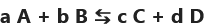When a non-total reaction reaches its final state, the quantities of matter remain constant because the chemical species are consumed and produced at the same rate  :

The final state of a non-total reaction corresponds to an equilibrium during which the rate of disappearance of each chemical species also corresponds to its rate of formation.

The final state of a non-total reaction is a so-called "  dynamic  " equilibrium, the system is not fixed, the quantities of matter remain globally constant but the two transformations (in one direction and in the opposite direction) continue to take place .

## Progress rate of a reaction

The progress rate is a quantity defined for a non-total reaction.

It is noted “ τ  ” (small Greek letter “tau”) and has no unit .

It is defined as the ratio of the progress of the reaction to the final state "x  " by the maximum progress of the reaction "x max  ":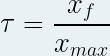with x f and x max in mole ( mol ) and unitless τ.

Interpretation of the reaction rate value:

• If τ = 0 then the reaction does not take place in the direction considered or is complete in the opposite direction

• If 0 < τ < 0.5 then the reactants remain in the majority compared to the products in the final state

• If τ = 0.5 then half of the products have been consumed in the final state

• If 0.5 < τ < 1 then the products are in the majority compared to the reactants in the final state

• If τ = 1 then the reaction is complete.

## Reaction Quotient Qr

If a chemical system (at equilibrium or not) is the seat of a non-total reaction described by an equation of the following form:Then the reaction quotient is the quantity noted "Q r  ", without unit defined at any time of the chemical transformation by the following relationship: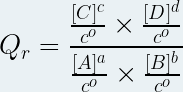where :

• Qr is the reaction quotient (no unit)

• [A], [B] are the molar concentrations of the reactants in mole per liter ( mol .L -1 )

• [C], [D] are the molar concentrations of the products in mole per liter ( mol .L -1 )

• a, b, c, d are the respective stoichiometric coefficients of the chemical species A, B, C and D

• c° is the standard molar concentration (c° = 1 mol .L -1 )

Taking into account that the standard concentration has a value of one mole per liter, we can simplify the expression of the reaction quotient: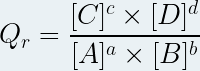Only the dissolved chemical species intervene in the expression of the reaction quotient (the insoluble solids , the solvent do not intervene).

## Equilibrium constant K

The equilibrium constant of a non-total reaction is noted “K” and corresponds to the value taken by the reaction quotient when equilibrium is reached.

If the equilibrium constant takes a value greater than 10 4 (10000) then we can consider that the reaction is complete in the forward direction.

## Direction of spontaneous evolution of a chemical system

It is possible to predict the direction in which a chemical system is changing by comparing its reaction quotient to its equilibrium constant.

By definition, if Qr = K then the chemical system is at equilibrium (it no longer evolves, the concentrations remain constant)

If Qr < K (the reaction quotient is less than the equilibrium constant) then the system evolves in the forward direction (the concentration of the reactants decreases and that of the products increases)

If Qr > K (the reaction quotient is greater than the equilibrium constant) then the system evolves in the non-direct direction (the concentration of the reactants increases and that of the products decreases)

## Operating principle of an electrochemical cell

An electrochemical cell always corresponds to the association of two half-cells  :

A half-cell associates the two members of the same oxidizing-reducing couple .

For example, a half-cell can combine metallic copper (Cu) and copper ions (Cu 2+ ) belonging to the Cu 2+ / Cu pair.

These two couples are not brought into contact because otherwise there would be a direct reaction between them, however they are connected:

• by an element providing ionic conduction between the two half-cells (often a salt bridge)

• as well as by the external electrical circuit

Each half-cell is the seat of a half-reaction:

• The half-cell comprising the most oxidizing couple is the seat of a reduction (gain of electrons ), it constitutes the cathode .

• The half-cell comprising the most reducing torque is the site of oxidation (loss of electrons ), it constitutes the anode .

Electrons flow in the electrical circuit from the anode to the cathode.

To ensure the electrical neutrality of the half-cells, the ions circulate through the salt bridge:

• The (positive) cations migrate to the cathode (where the negative electrons end up)

• The ( negative ) anions migrate to the anode (which the negative electrons leave)

Example: the copper-zinc battery

A copper-zinc battery is made up of the association:

• of a half-cell involving the Cu 2+ /Cu couple

• of a half-cell involving the couple Zn 2+ /Zn

The half-cell corresponding to the Cu 2+ /Cu pair can be obtained by immersing a sheet of metallic copper in a copper II sulphate solution.

Cu 2+ being more oxidizing than Zn 2+ this half-cell is the seat of the reduction of the copper ions according to the following half equation:

Cu 2+ + 2e- → Cu

This half-cell constitutes the cathode of the cell

The half-cell corresponding to the Zn2+/Zn pair can be obtained by immersing a sheet of metallic zinc in a zinc sulphate solution.

This half-cell is the seat of zinc oxidation according to the following half-equation:

Zn → Zn 2+ + 2e –

This half-cell constitutes the anode of the cell

When this cell works, electrons circulate (in the circuit outside the cell) from the zinc anode to the copper cathode, while within the cell the cations migrate to the copper cathode and the anions to the copper anode. zinc.

## Electrical capacity of a battery

Definition

The electrical capacity of a battery, generally denoted Q max , corresponds to the total electrical charge (in coulombs) that this battery is capable of circulating in an external electrical circuit .

It can be expressed from the amount of matter of electrons that the battery is able to circulate from the following formula:

max = n e x F

In this formula:

• max is the electric capacity of the battery in coulomb (C)

• e is the amount of total electron matter the cell can circulate in mole ( mol )

• F is Faraday's constant which corresponds to the electric charge of one mole of electrons (F = 6.02.10 23 x 1.6.10 -19 therefore F = 9.65.10 4 C. mol -1 )

The electrical capacity corresponds to the electrical charge exchanged by the oxidant and the reducer of the cell until the state of equilibrium (Qr = K) is reached.

The electrical capacity is often expressed in another unit: the Ampere-hour (symbol Ah or Ah )

The ampere-hour is defined as the electric charge exchanged when an electric current of intensity I = 1 A circulates for a period of one hour.

From this definition follows the following equivalence:

1 Ah = 3600 C

Therefore, we can convert coulombs to ampere-hours using the relationship:

Charge in Amp-hour = (Charge in Coulomb)/3600

Conversely :

Charge in Coulomb = (Charge in Ampere-hour) x 3600

The operating time of a battery depends on its electrical capacity (and therefore on the quantity of reagents it contains) but also on the intensity of the electrical current that it supplies to the external circuit .

Given that the intensity of the current corresponds to the flow of electric charge expressed by the relation: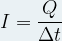where :

• I is the intensity of the electric current flowing in Ampere (A)

• Q is the electric charge in Coulomb (C)

• Δt is the time during which the current flows in second (s)

Then we can express the maximum operating time Δt max of a battery thanks to the following relationship: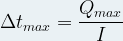Science class Mechanics lessons Water Water on Earth Changes of state in the nature: the water cycle Water in human body Test for water Properties of water in different states States of matter States of matter and its changes Boiling water Water: freezing and melting Changes of state: mass and volume Molecules in different states of matter Mixtures and solutions Heterogeneous mixtures Homogeneous mixtures Decantation Centrifugation Filtration Vaporization of water Distillation Chromatography Dissolving a solid in water Conservation of mass on dissolving Miscibility in water Mass and volume Volume and its units Measuring volume with a graduated cylinder Mass and its units Measuring the mass of a liquid Mass of a liter of water Volumetric mass density Air and atmosphere Earth's atmosphere protect us Pressure Atoms and molecules Molecules Molecules in mixtures and pure substances Molecules and states of matter Atoms Composition of molecules Constituents of the atoms The electrical neutrality of the atom Combustions Basics of combustion The combustion of carbon The combustion of butane Atoms and chemical reactions Chemical reactions Chemical equations Law of conservation of mass Chemical synthesis Metals Most common metals How to distinguish metals ? Corrosion of metals Electrical conductivity of solid materials Electrons and free electrons Electric current in metals Ions The conductivity of aqueous solutions Aqueous solutions and ions The direction of movement of ions Formation of ions Tests for ions Acidic and basic solutions pH of aqueous solutions Ions in acidic and basic solutions Dilution of acids and bases Composition of hydrochloric acid Chemical reaction between iron and hydrochloric acid Electrochemical cells and chemical energy Chemical reaction beteween a copper sulphate solution and zinc Copper sulfate and zinc battery Basics of electrochemical cell

©2021 Physics and chemistry